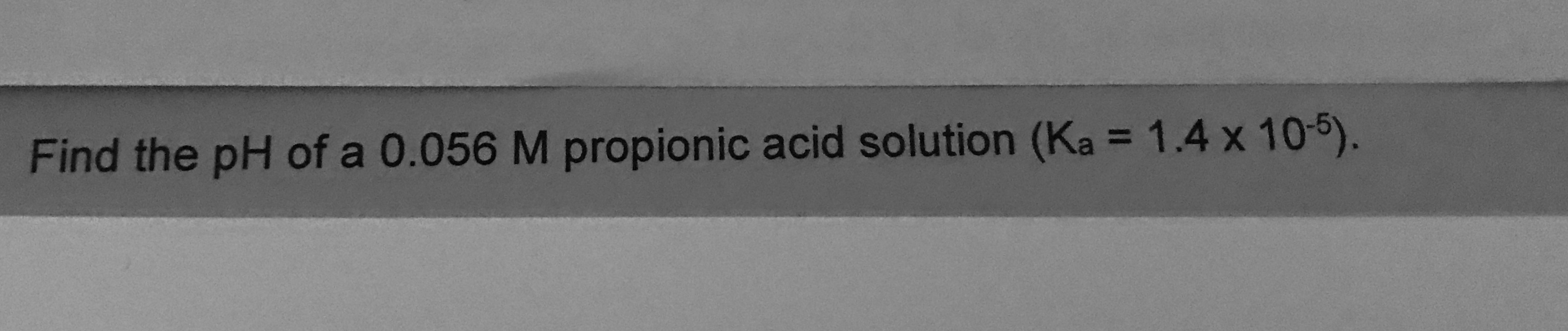# Find the pH of a 0.056 M propionic acid solution (Ka = 1.4 x 10-5).%3D

Question
75 viewshelp_outlineImage TranscriptioncloseFind the pH of a 0.056 M propionic acid solution (Ka = 1.4 x 10-5). %3D fullscreen
check_circle

Step 1

The reaction of propionic acid with water is written as follows:

CH3CH2COOH(aq) + H2O (l)→ CH3CH2COO-(aq) + H3O+(aq)

The ICE table for the above reaction is shown below.

Step 2

Write the acid dissociation constant for the above reaction as written in equation (1).

Now, substitute 1.4×10-5 for Ka, x for CH3CH2COO-and H3O+ and 0.056 - x for CH3CH2COOH as shown in equation  (2).

Step 3

Neglecting x in the denominator as shown in equation  (3),

&nbs...

### Want to see the full answer?

See Solution

#### Want to see this answer and more?

Solutions are written by subject experts who are available 24/7. Questions are typically answered within 1 hour.*

See Solution
*Response times may vary by subject and question.
Tagged in